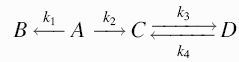Search BioKin.comKinetics with Mathematica
Generate Mathematica code for deriving simple rate lawsThis tool will generate Mathematica code to derive rate laws for simple unimolecular (uniformly first order) reaction mechanisms.

• This tool does not handle bimolecular steps.
• The only type reactions allowed are unimolecular.
• Example: X --> Y or X <==> Y.
• Mathematica will not handle more than four reacting species.

#### ExampleThe above mechanism needs to be represented as follows:

```A ---> B   :   k1
A ---> C   :   k2
C <==> D   :   k3  k4
```
##### References
The example is taken from Chapter 2 (pp. 41-45) of the following book:
```	Korobov, V. I. and Ochkov, V. F. (2011)
"Chemical Kinetics with Mathcad and Maple"
Springer-Verlag, Wien

344 p., ISBN: 978-3-7091-0530-6
```www.biokin.com/tools/mathekin.html